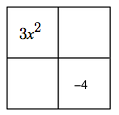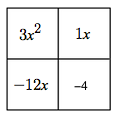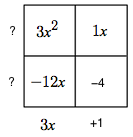### Home > CCA2 > Chapter Ch5 > Lesson 5.2.3 > Problem5-91

5-91.

Factor each expression below.

1. $x^2+8x$

Look for a greatest common factor.

2. $x^2y^2−81z^2$

This is the difference of squares:

$(xy)^2 - (9z)^2$

If you need more help, look up difference of squares in the glossary or find your class work from Lesson 3.1.2.

3. $2x^2+14x−16$

Always start by looking for a greatest common factor.

Can you factor the polynomial further?

4. $3x^2−11x−4$

Use a generic rectangle. Remember that the products of the diagonals must be equal.

. Image of 2 by 2 rectangle.

Start by filling in the first and last terms.

Added to interior labeled, top left, 3, x squared, bottom right, negative 4.

What two numbers multiply to $−12$ and add to $−11$?  Added to rectangle, interior labels, top right, 1, x, bottom left, negative 12, x.

Find the greatest common factor for each row and column.Added to rectangle, labels, left edge, top & bottom, each with question mark, bottom edge, left, 3, x, right, + 1.

$3x^2-11x-4=(3x+1)(?+?)$